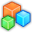# Microsoft Small BasicProgram Listing: CVS185-0
' SmallBasic Version 1.2
' Program: CartesianToPolar
' Changelog:
' Author: Pappa Lapub
' Website: https://social.msdn.microsoft.com/Forums/en-US/95706095-0984-40d7-897f-0ec14ed58039/small-basic-subroutine-library-project
' ImportURL: http://smallbasic.com/program/?CVS185-0
' SBOnline: http://smallbasic-publicwebsite-dev.azurewebsites.net/Program/view.aspx?
' Extension: LitDev
' Comment:
'
' Variables:
' ToDo:
' ==============================================================================
using an angle of 30°, tan(30°) = 1/sqrt(3)
x = 100
y = 100 / Math.SquareRoot(3)

_r = ""
_a = ""
path = Program.Directory +"\Math_Functions.sbsub" ' is a renamed SB-Exe with Sub Routines
incl = LDCall.Include(path)
If incl <> "" Then
LDCall.CallInclude(incl, "Math_CartesianToPolar") ' Name of the Sub to call
' now return values should be in _r and _a
Else
Program.End()
EndIf

'' TEST

'' call as function (within EntryAssembly)
args = ""
res = ""
res = LDCall.Function("CartesianToPolar", "1="+ _r +";2="+ _a +";") '' or ...
'res = LDCall.Function2("CartesianToPolar", _r,_a)

TextWindow.WriteLine("x= "+ x)
TextWindow.WriteLine("y= "+ y)
TextWindow.WriteLine("")

TextWindow.WriteLine("r= "+ _r)
TextWindow.WriteLine(" (Test 'LDMath.Convert2Radial' = "+ polar +")")
TextWindow.WriteLine(" rFnc= "+ res)

TextWindow.WriteLine("a= "+ _a)
TextWindow.WriteLine(" (Test 'LDMath.Convert2Radial' = "+ polar +")")
TextWindow.WriteLine(" aFnc= "+ res)
TextWindow.WriteLine("")

' //////////////// FUNCTIONs /////////////////
Sub CartesianToPolar
return = _r
return = _a
EndSub